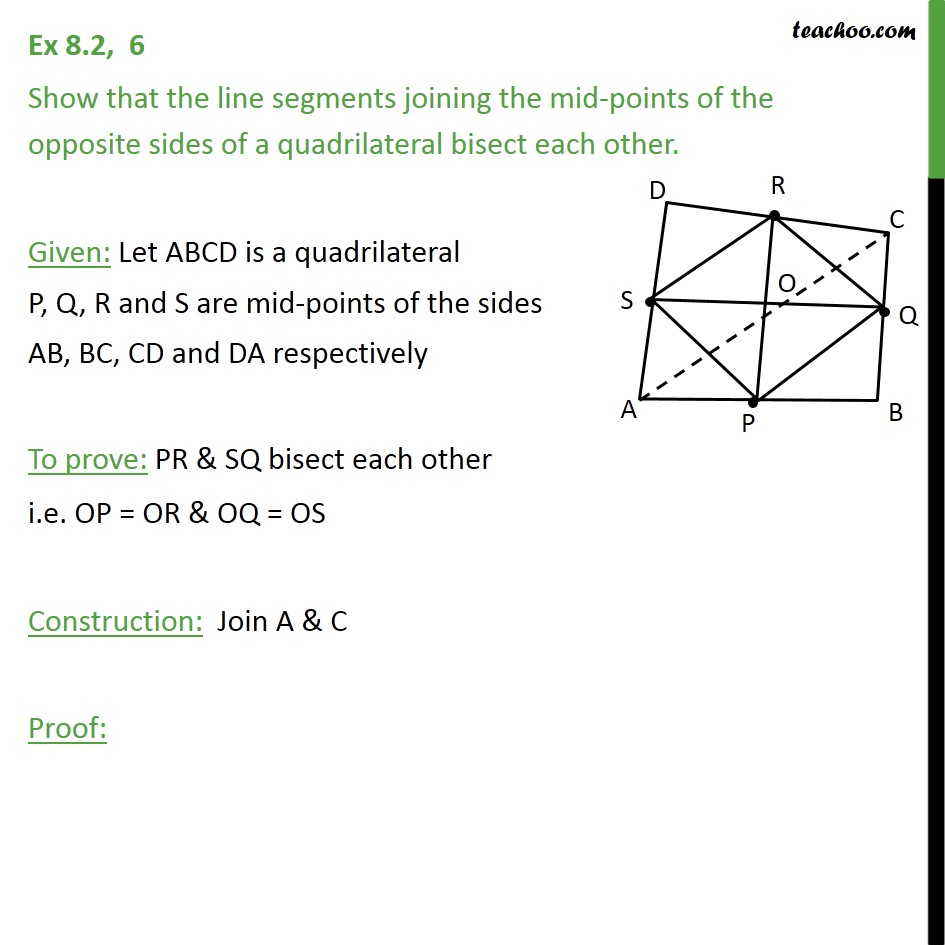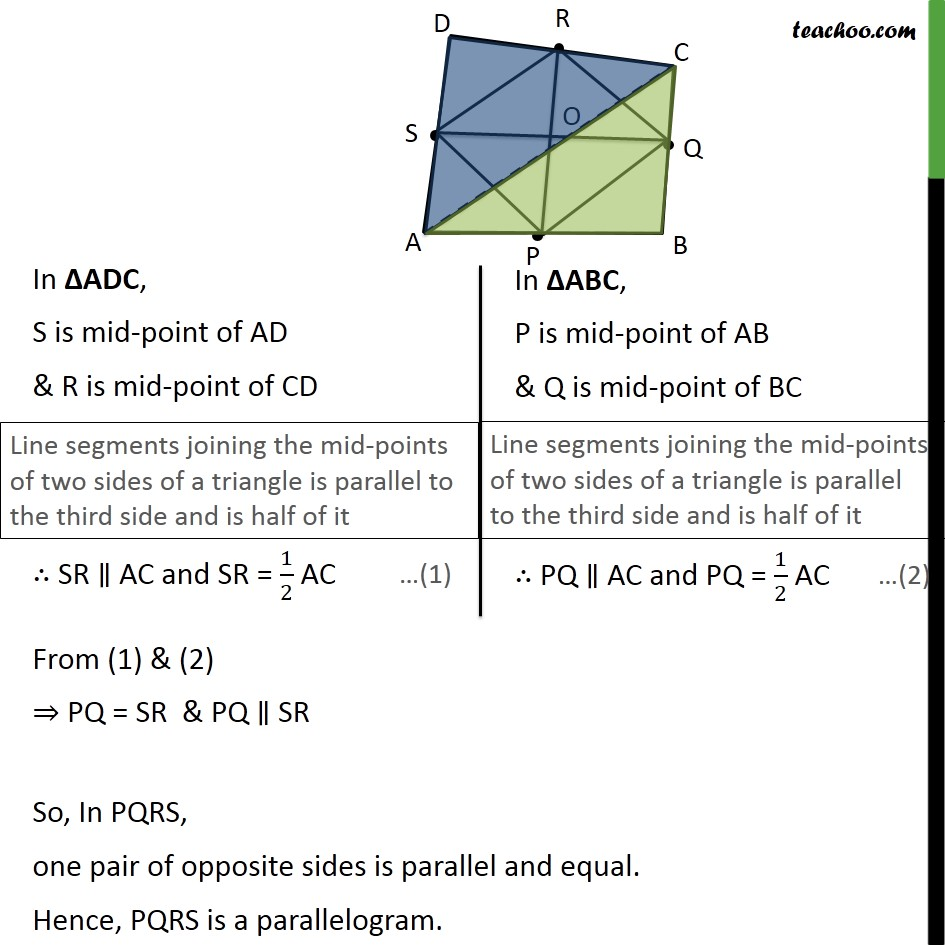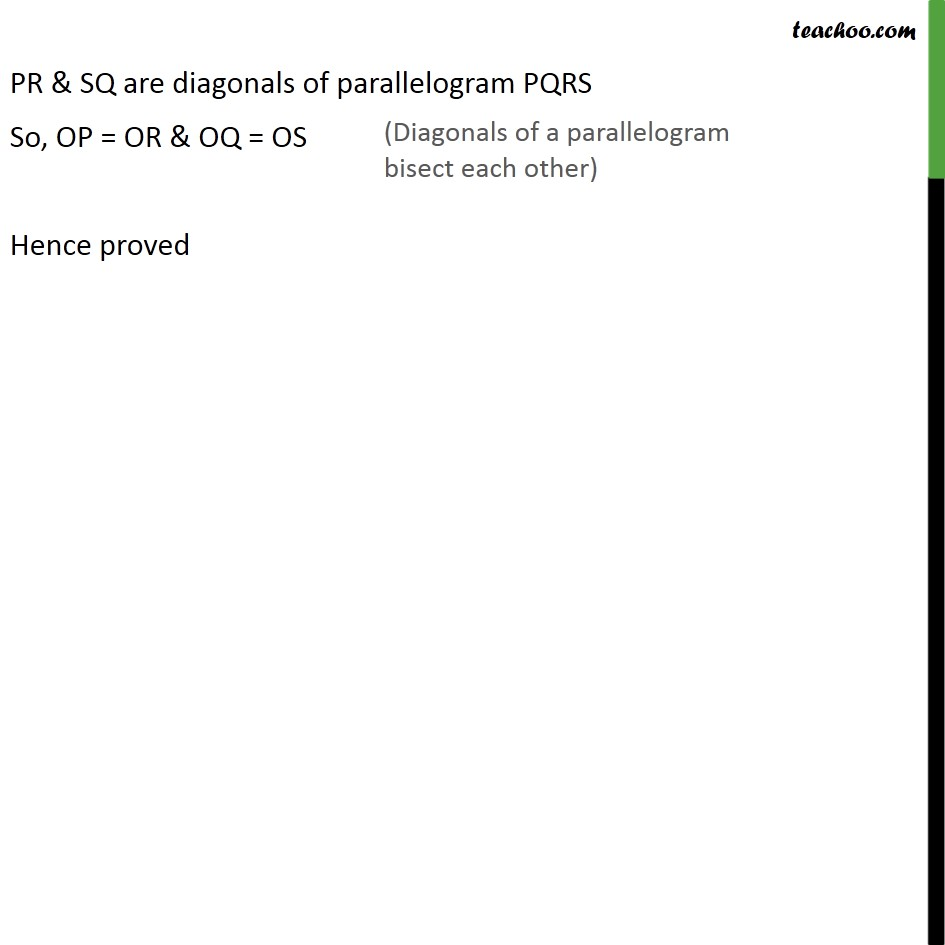Subscribe to our Youtube Channel - https://you.tube/teachoo

1. Chapter 8 Class 9 Quadrilaterals
2. Serial order wise
3. Ex 8.2

Transcript

Ex 8.2, 6 Show that the line segments joining the mid-points of the opposite sides of a quadrilateral bisect each other. Given: Let ABCD is a quadrilateral P, Q, R and S are mid-points of the sides AB, BC, CD and DA respectively To prove: PR & SQ bisect each other i.e. OP = OR & OQ = OS Construction: Join A & C Proof: PR & SQ are diagonals of parallelogram PQRS So, OP = OR & OQ = OS Hence proved

Ex 8.2